# Programming Exercises in C++

## Programming Exercises in C++

In this article, we will see some programs which will take some input from users, process the input data, and show the output to the screen. Please read our previous article where we discussed the Roots of Quadratic Equations in C++ with Examples.

##### Area of Circle:

We will show you the program which calculates the area of a circle. We know the area of the circle is 3.14 * r * r. Let’s see the code part.

##### Area of Circle Code in C++ Language:
```#include <iostream>
using namespace std;
int main()
{
cout << "Enter Radius of Circle: ";
cout << "Area is " << area;
return 0;
}
```
###### Output: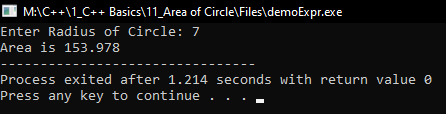##### Calculate Displacement:

Displacement is defined as the shortest path covered by a body. We have formula to calculate the Displacement: s = (v2 – u2) / 2a

##### Calculate Displacement Code in C++ Language:
```#include <iostream>
using namespace std;
int main()
{
int u, v, a;
float disp;
cout << "Enter u, v and a: ";
cin >> u >> v >> a;
disp = (v * v - u * u) / (2 * a);
cout << "Displacement is " << disp;
return 0;
}
```
###### Output: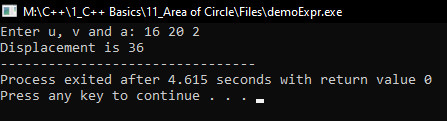##### Find Distance Between Two Points Program in C++ Language:
```#include <iostream>
using namespace std;

int main(){
float s, d, t;

cout << "Enter s (km/h) and t (h): ";
cin >> s >> t;

d = s * t;

cout << "Distance is: " << d << " km" << endl;
return 0;
}
```
###### Output: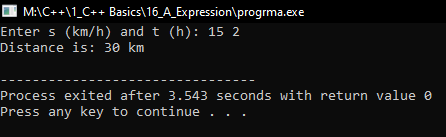##### Simple Interest Program in C++ Language:
```#include <iostream>
using namespace std;

int main(){
float p, r, t;
float SI, A;

cout << "Enter p, r, t: " << endl;
cin >> p >> r >> t;

SI = p * r * t / 100;
A = p + SI;

cout << "Interest is " << SI << endl;
cout << "Total value: " << A << endl;
return 0;
}
```
###### Output: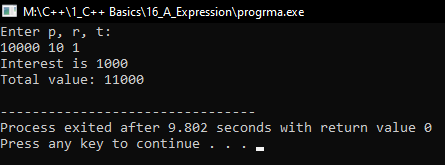##### Volume of Cylinder Program in C++ Language:
```#include <iostream>
using namespace std;

int main(){
float r, h, v;

cout << "Enter r and h: " << endl;
cin >> r >> h;

v = 3.14 * r * r * h;

cout << "Volume of cylinder is " << v << endl;
return 0;
}
```
###### Output: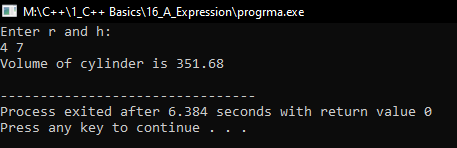##### Distance (using speed and time) Program in C++ Language:
```#include <iostream>
using namespace std;

int main(){
float s, d, t;

cout << "Enter s (km/h) and t (h): ";
cin >> s >> t;

d = s * t;

cout << "Distance is: " << d << " km" << endl;
return 0;
}
```
###### Output:##### Salary Program in C++ Language:
```#include <iostream>
using namespace std;

int main(){
float basic;
float percentAllow;
float percentDeduct;
float netSalary;

cout << "Enter Basic Salary: ";
cin >> basic;
cout << "Enter percet of Allowences: ";
cin >> percentAllow;
cout << "Enter percent of Deductions: ";
cin >> percentDeduct;

netSalary = basic + basic * percentAllow / 100 - basic * percentDeduct /100;

cout << "Net Salary is: " << netSalary << endl;

return 0;
}
```
###### Output: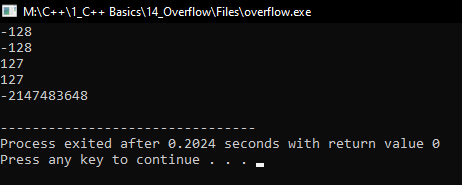In the next article, I am going to discuss Compound Assignment Operator in C++ with Examples. Here, in this article, I try to explain Programming Exercises in C++ with Examples and I hope you enjoy this Programming Exercises in C++ with Examples article.